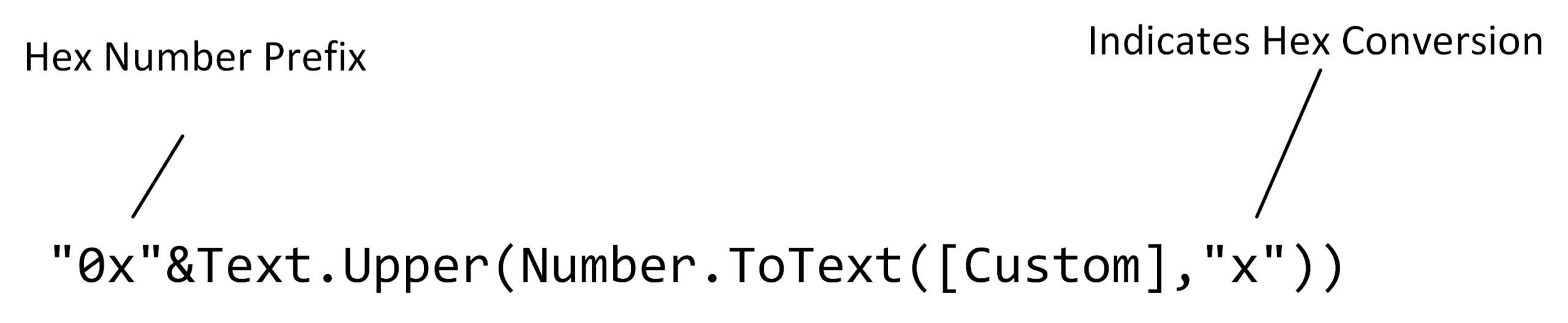# Converting a Binary Table To Hex With Power Query

Quote of the Day

Courage is not the absence of fear, but rather the assessment that something else is more important than fear.

## Introduction

I was recently given a specification for a Digital-to-Analog Converter (DAC) that contains a table of register settings that correspond to the DAC's output voltage. Each row of the table corresponds to one register setting and each register column corresponds to a register bit. Thus, each table cell corresponds to a single bit – a 1 or 0. A simplified version of this table is shown in Figure 1 – a simplified version of the much larger table I was working with.

I wanted to convert the table's binary settings to hexadecimal for ease of programming. As I looked at the table, it became apparent that converting these binary values to hexadecimal will allow me to showcase some of Power Query's list processing capabilities.

In this exercise, I will show to use Power Query to

• Convert the binary columns to a decimal value
• Convert the decimal value to hexadecimal

I like this example because it is short and easy to follow. I use this example in my Excel tutoring to illustrate the use of lists and records. My workbook containing the m-code is here. Please refer to the workbook for the details. Below, I discuss a couple of points that people sometimes find confusing.

## Background

### Convert Binary to Decimal

Before going forward, it is important to understand the data types associated with a Power Query table:

• Columns are lists
• Rows are records
• You address a subset of the cells in a single table row using the syntax
table_name{row_number}[[col1], [col2], ..., [coln]]
• This subset will have a data type of record.

The following formula is used in the Excel workbook to generate a decimal number from the individual binary digits.### Convert Binary to Hex

There are a couple of ways to perform binary-to-hex conversion. The approach here uses Power Query's Number.ToText function to perform the conversion.## Result

The workbook outputs the following table.I have used this approach for converting numerous binary tables to hex form. This approach also provides a nice illustration of how to use the list.accumulate function.

This entry was posted in Excel. Bookmark the permalink.

### 2 Responses to Converting a Binary Table To Hex With Power Query

1.Joel Lagerquist says:

People who write the documentation try to be precise. It can become confusing though.

Why not just say. The register value divided by 3 is the voltage.

Saying what the value of a bit is seems confusing. I have seen similar things on ADCs.

I know you are using it as an example for using spreadsheet functions.

•mathscinotes says:

Hi Joel,

Great to hear from you! Hopefully, the new job is going well.

This is a real example from a product spec. I would much rather just work with formulas. However, it is what it is. I see tables like this being used all the time when a simple formula would be better. In this case, I needed to use the table in a document, so I used Power Query for converting the binary columns to hexadecimal, which I prefer for coding.

biegert